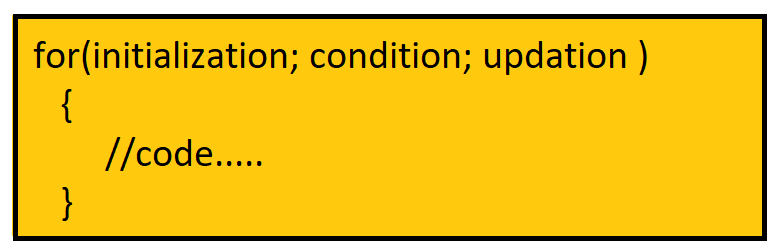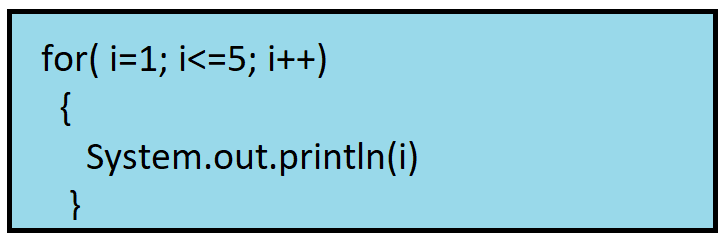# For loop in Java

### What is a loop?

Loops help to execute a set of statements/code blocks repeatedly many times till the condition is met.

### Why do we need loops?

Let’s say we want to add 2 numbers which can be easily done by number1+number2

But what if there is a requirement to add 100 numbers? Well, here loops play an important role

As mentioned in the definition, the code block in the loop will run 100 times hence we’ll get the desired output, So loops are very efficient to execute the same instructions again and again.

Java Programming language has different types of loops:

1. For loop
2. While loop
3. Do while loop
4. For each loop

In this article, we will learn about the For loop.

### For loop:

For loop executes the set of code repeatedly for a specific number of times, if the programmer knows how many times the loop has to be executed then For loop is best in that case.

Syntax:1. Initialization → From which value the loop has to start.
2. Condition     → When the loop should stop
3. Updation     → Incrementation or decrementation

How does it work?

Example 1: Program to print the first 5 natural numbers.1. Initially, the i value will be 1 and i is <= 5, So i (1)  will be printed and i will get updated to 2
2. Now i value is 2, 2<=5 so i (2) will be printed and i will get updated to 3
3. Now i value is 3, 3<=5 so i (3) will be printed and i will get updated to 4
4. Now i value is 4, 4<=5 so i (4) will be printed and i will get updated to 5
5. Now i value is 5, 5<=5 so i (5) will be printed and i will get updated to 6
6. Now i value is 6, 6<=5 (false) so the loop will terminate, this is how it repeatedly executes code till the condition is met.

Code:

## Java Code

``````public class TUF {
public static void main(String[] args) {
//Program to demonstrate for loop

// initialization; condition; increment
for (int i=1;i<=5;i++){
System.out.print(i+" ");
}
}
}
``````

Output: 1 2 3 4 5

Example 2: Program to print a table of a number, say 5

Code:

## Java Code

``````public class TUF {
public static void main(String[] args) {
//Program to print table using for loop
int n = 5;
// initialization; condition; increment
for (int i=1;i<=10;i++){
System.out.println(n+"*"+i+" - " +n*i);
}
}
}
``````

Output:

5*1 – 5

5*2 – 10

5*3 – 15

5*4 – 20

5*5 – 25

5*6 – 30

5*7 – 35

5*8 – 40

5*9 – 45

5*10 – 50

Special thanks to Sai bargav Nellepalli for contributing to this article on takeUforward. If you also wish to share your knowledge with the takeUforward fam, please check out this article